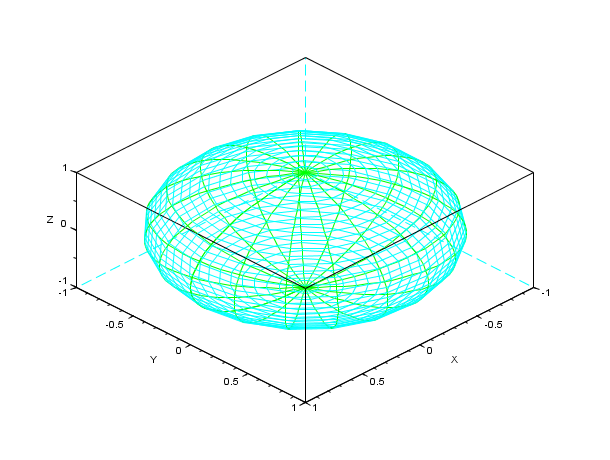Change language to:
English - 日本語 - Português - Русский

See the recommended documentation of this function

Aide de Scilab >> Graphiques > 3d_plot > plot3d3

# plot3d3

mesh plot surface defined by rectangular facets

### Syntax

```plot3d3(X,Y,Z [,vect,theta,alpha,leg,flag,ebox])
plot3d3(X,Y,Z, <opt_args>)```

### Arguments

X, Y, Z:

3 real matrices defining a data structure.

vect

a real vector.

<opt_args>

This represents a sequence of statements `key1=value1, key2=value2`,... where `key1`, `key2,...` can be one of the following: theta, alpha ,leg,flag,ebox (see definition below).

theta, alpha

real values giving in degree the spherical coordinates of the observation point.

leg

string defining the labels for each axis with @ as a field separator, for example "X@Y@Z".

flag

a real vector of size four. `flag=[vertical_color, horizontal_color,type,box]`.

vertical_color

an integer (surface color), default is 3.

Colormap index defining the color used to draw vertical edges.

horizontal_color

an integer (surface color), default is 4.

Colormap index defining the color used to draw horizontal edges.

type

an integer (scaling) default is 2.

type=0:

the plot is made using the current 3D scaling (set by a previous call to `param3d`, `plot3d`, `contour` or `plot3d1`).

type=1:

rescales automatically 3d boxes with extreme aspect ratios, the boundaries are specified by the value of the optional argument `ebox`.

type=2:

rescales automatically 3d boxes with extreme aspect ratios, the boundaries are computed using the given data.

type=3:

3d isometric with box bounds given by optional `ebox`, similarily to `type=1`.

type=4:

3d isometric bounds derived from the data, to similarily`type=2`.

type=5:

3d expanded isometric bounds with box bounds given by optional `ebox`, similarily to `type=1`.

type=6:

3d expanded isometric bounds derived from the data, similarily to `type=2`.

Note that axes boundaries can be customized through the axes entity properties (see axes_properties).

box

an integer (frame around the plot), default is 4.

box=0:

nothing is drawn around the plot.

box=1:

unimplemented (like box=0).

box=2:

only the axes behind the surface are drawn.

box=3:

a box surrounding the surface is drawn and captions are added.

box=4:

a box surrounding the surface is drawn, captions and axes are added.

Note that axes aspect can also be customized through the axes entity properties (see axes_properties).

ebox

It specifies the boundaries of the plot as the vector `[xmin,xmax,ymin,ymax,zmin,zmax]`. This argument is used together with `type` in `flag` : if it is set to `1`, `3` or `5` (see above to see the corresponding behaviour). If `flag` is missing, `ebox` is not taken into account. Note that, when specified, the `ebox` argument acts on the `data_bounds` field that can also be reset through the axes entity properties (see axes_properties).

### Description

`plot3d3` performs a mesh plot of a surface defined by rectangular facets. (X,Y,Z) are three matrices which describe a surface. The surface is composed of four sided polygons.

The X-coordinates of a facet are given by X(i,j), X(i+1,j), X(i+1,j+1) and X(i,j+1). Similarly Y and Z matrices contain Y and Z-coordinates.

The `vect` vector is used when multiple surfaces are coded in the same (X,Y,Z) matrices. `vect(j)` gives the line at which the coding of the jth surface begins. See `plot3d2` for a full description. As a mesh plot, the only available property you can edit is the `visible` option (see axes_properties).

### Examples

```u = linspace(-%pi/2,%pi/2,40);
v = linspace(0,2*%pi,20);
X = cos(u)'*cos(v);
Y = cos(u)'*sin(v);
Z = sin(u)'*ones(v);
plot3d3(X,Y,Z);
e=gce(); // get the current entity
e.visible='off';
e.visible='on'; // back to the mesh view```